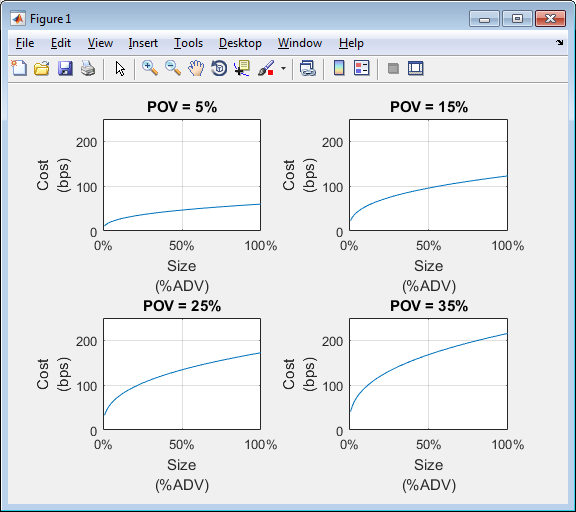# costCurves

Estimate market-impact cost of order execution

## Syntax

``cc = costCurves(k,trade,tradeQuantity,tqRange,tradeStrategy,tsRange)``

## Description

example

````cc = costCurves(k,trade,tradeQuantity,tqRange,tradeStrategy,tsRange)` returns the market-impact costs of order execution using: Kissell Research Group (KRG) transaction cost analysis object `k`Trade data `trade`Trade quantity `tradeQuantity` with a range of values `tqRange`Trade strategy `tradeStrategy` with a range of values `tsRange` ```

## Examples

collapse all

Retrieve the market impact data from the KRG FTP site. Connect to the FTP site using the `ftp` function with a user name and password. Navigate to the `MI_Parameters` folder and retrieve the market impact data in the `MI_Encrypted_Parameters.csv` file. `miData` contains the encrypted market impact date, code, and parameters.

```f = ftp('ftp.kissellresearch.com','username','pwd'); mget(f,'MI_Encrypted_Parameters.csv'); miData = readtable('MI_Encrypted_Parameters.csv','delimiter', ... ',','ReadRowNames',false,'ReadVariableNames',true);```

Create a Kissell Research Group transaction cost analysis object `k`.

`k = krg(miData);`

Load the example data from the file `KRGExampleData.mat`, which is included with the Datafeed Toolbox™.

`load KRGExampleData`

The variable `TradeData` appears in the MATLAB® workspace.

`TradeData` contains these variables:

• Stock symbol

• Stock price

• Average daily volume

• Volatility

For a description of the example data, see Kissell Research Group Data Sets.

Estimate market-impact costs with the trade quantity `'Size'` and strategy `'POV'`. Specify the trade quantity range with increments of 0.01 by starting from 0.01 and ending at one. Specify the trade strategy range with increments of 0.05 by starting from 0.05 and ending at 0.5.

`cc = costCurves(k,TradeData,'Size',(0.01:0.01:1),'POV',(0.05:0.05:0.5));`

Display the first three rows of market-impact cost data.

`cc(1:3,:)`
```ans = Symbol Size Shares Dollars POV TradeTime Cost_BP Cost_DollarsPerShare Cost_Dollars ______ ____ _________ __________ ____ _________ _______ ____________________ ____________ 'AAL' 0.01 114764.24 6251208.50 0.05 0.19 11.42 0.06 7139.93 'AAL' 0.01 114764.24 6251208.50 0.10 0.09 17.93 0.10 11210.76 'AAL' 0.01 114764.24 6251208.50 0.15 0.06 23.42 0.13 14637.37 ```

The market-impact cost data contains:

• Stock symbol

• Size

• Number of shares in the transaction

• Dollar amount of the transaction

• Percentage of volume to complete the transaction

• Trade time to complete the transaction in percentage of the day

• Market-impact cost in basis points

• Market-impact cost in dollars per share

• Market-impact cost in dollars

Display cost curves for the first stock for these percentage of volume rates: 5%, 15%, 25%, and 35%.

```figure subplot(2,2,1) plot(cc.Size(1:10:1000)*100,cc.Cost_BP(1:10:1000)) grid on axis([0 100 0 250]) xlabel({'Size','(%ADV)'}) ylabel({'Cost','(bps)'}) title('POV = 5%') a = gca; a.XAxis.TickLabelFormat = '%g%%'; subplot(2,2,2) plot(cc.Size(3:10:1000)*100,cc.Cost_BP(3:10:1000)) grid on axis([0 100 0 250]) xlabel({'Size','(%ADV)'}) ylabel({'Cost','(bps)'}) title('POV = 15%') b = gca; b.XAxis.TickLabelFormat = '%g%%'; subplot(2,2,3) plot(cc.Size(5:10:1000)*100,cc.Cost_BP(5:10:1000)) grid on axis([0 100 0 250]) xlabel({'Size','(%ADV)'}) ylabel({'Cost','(bps)'}) title('POV = 25%') c = gca; c.XAxis.TickLabelFormat = '%g%%'; subplot(2,2,4) plot(cc.Size(7:10:1000)*100,cc.Cost_BP(7:10:1000)) grid on axis([0 100 0 250]) xlabel({'Size','(%ADV)'}) ylabel({'Cost','(bps)'}) title('POV = 35%') d = gca; d.XAxis.TickLabelFormat = '%g%%';```This figure demonstrates how fast to trade a specific order size within a price level.

## Input Arguments

collapse all

Transaction cost analysis, specified as a KRG object created using `krg`.

Trade data that describes the stocks in the transaction, specified as a table or structure. `trade` must contain these variable or field names.

Variable or Field NameDescription

`Symbol`

Stock symbol

`Price`

Stock price

`ADV`

Average daily volume

`Volatility`

Volatility

Example: ```trade = table({'XYZ'},100.00,860000,0.27,'VariableNames',{'Symbol' 'Price' 'ADV' 'Volatility'})```

Example: ```trade = struct('Symbol','XYZ','Price',100.00,'ADV',860000,'Volatility',0.27)```

These examples do not represent real market data.

Data Types: `struct` | `table`

Trade quantity, specified as one of these values.

`'Size'`

Shares in the transaction, which is a percentage of average daily trading volume

`'Shares'`

Number of shares in the transaction

`'Dollars'`

Total value of the transaction

Trade quantity range, specified as a vector. `costCurves` uses these values with the trade strategy range values to estimate market-impact costs for different quantities and strategies.

Example: `'Size',(0.01:0.01:1)` specifies a trade quantity range with increments of 0.01 starting from 0.01 and ending at one

Data Types: `double`

Trade strategy, specified as one of these values.

`'POV'`

Percentage of volume

`'TradeTime'`

Trade time in percentage of the day

Trade strategy range, specified as a vector. `costCurves` uses these values with the trade quantity range values to estimate market-impact costs for different quantities and strategies.

Example: `'POV',(0.05:0.05:0.5)` specifies a trade strategy range with increments of 0.05 starting from 0.05 and ending at 0.5

Data Types: `double`

## Output Arguments

collapse all

Cost curves, returned as a table or structure with these variable names or fields.

Variable or Field Name Description

`Symbol`

Stock symbol

`Size`

Shares in a transaction in percentage of average daily trading volume

`Shares`

Number of shares in the transaction

`Dollars`

Dollar amount of the transaction

`POV`

Percentage of volume to complete the transaction

`TradeTime`

Trade time to complete the transaction in percentage of the day

`Cost_BP`

Market-impact cost of the transaction in basis points

`Cost_DollarsPerShare`

Market-impact cost of the transaction in dollars per share

`Cost_Dollars`

Market-impact cost of the transaction in dollars

## Tips

• For details about the calculations, contact Kissell Research Group.

 Kissell, Robert. “A Practical Framework for Transaction Cost Analysis.” Journal of Trading. Vol. 3, Number 2, Summer 2008, pp. 29–37.

 Kissell, Robert. “Algorithmic Trading Strategies.” Ph.D. Thesis. Fordham University, May 2006.

 Kissell, Robert. “TCA in the Investment Process: An Overview.” Journal of Index Investing. Vol. 2, Number 1, Summer 2011, pp. 60–64.

 Kissell, Robert. The Science of Algorithmic Trading and Portfolio Management. Cambridge, MA: Elsevier/Academic Press, 2013.

 Kissell, Robert, and Morton Glantz. Optimal Trading Strategies. New York, NY: AMACOM, Inc., 2003.